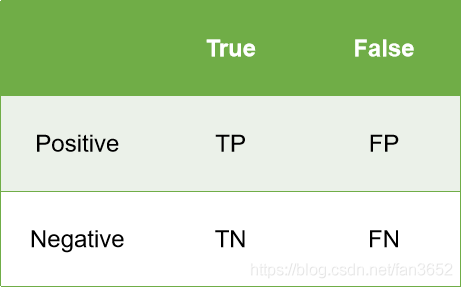40 篇文章 0 订阅

# 1.逻辑回归相比线性回归，有何异同？

y = f ( x ) = a x + b y=f(x)=ax+b

1.逻辑回归解决的是分类问题，线性回归解决的是回归问题，这是两者最本质的区别

2.逻辑回归中因变量是离散的，而线性回归中因变量是连续的这是两者最大的区别

3在自变量和超参数确定的情况下逻辑回归可看作广义的线性模型在因变量下服从二元分布的一个特殊情况

4.使用最小二乘法求解线性回归时我们认为因变量服从正态分布

1.二者在求解超参数的过程中都使用梯度下降的方法

2.他们两个都使用了极大似然估计来对训练样本进行建模。线性回归使用最小二乘法实际上就应该是极大似然估计的一个简化，而逻辑回归中也是通过对似然函数去学习才得到的最佳参数 θ 。

# 2.回归问题常用的性能度量指标

SSE、MSE、RMSE
SSE（残差平方和、和方差、误差平方和）：Sum of Squares due to Error

MSE(均方差、均方误差)：Mean Squared Error

RMSE(均方根误差、标准误差)：Root Mean Squared Error

S S E = ∑ i = 1 n ( y ^ i − y i ) 2 M S E = S S E n = 1 n ⋅ ∑ i = 1 n ( y ^ i − y i ) 2 R M S E = M S E = S S E / n = 1 n ⋅ ∑ i = 1 n ( y ^ i − y i ) 2 \begin{array}{l} S S E=\sum_{i=1}^{n}\left(\hat{y}_{i}-y_{i}\right)^{2} \\ M S E=\frac{S S E}{n}=\frac{1}{n} \cdot \sum_{i=1}^{n}\left(\hat{y}_{i}-y_{i}\right)^{2} \\ R M S E=\sqrt{M S E}=\sqrt{S S E / n}=\sqrt{\frac{1}{n} \cdot \sum_{i=1}^{n}\left(\hat{y}_{i}-y_{i}\right)^{2}} \end{array}

SSE、SSR、SST、R_square

SSE（残差平方和、和方差、误差平方和）：Sum of Squares due to Error

SSR(回归平方和)：Sum of Squares of the Regression

SST(总离差平方和)：Total Sum of Squares

R-square(决定系数)：Coefficient of Determination

S S E = ∑ i = 1 n ( y ^ i − y i ) 2 S S R = ∑ i = 1 n ( y ^ i − y ˉ ) 2 S S T = ∑ i = 1 n ( y i − y ˉ ) 2 S S T = S S E + S S R R 2 = S S R S S T = 1 − S S E S S T \begin{array}{l} S S E=\sum_{i=1}^{n}\left(\hat{y}_{i}-y_{i}\right)^{2} \\ S S R=\sum_{i=1}^{n}\left(\hat{y}_{i}-\bar{y}\right)^{2} \\ S S T=\sum_{i=1}^{n}\left(y_{i}-\bar{y}\right)^{2} \\ S S T=S S E+S S R \\ R^{2}=\frac{S S R}{S S T}=1-\frac{S S E}{S S T} \end{array}

MAE：直接计算模型输出与真实值之间的平均绝对误差
MAPE：不仅考虑预测值与真实值误差，还考虑了误差与真实值之间的比例。

M A E = 1 n ∑ i = 0 n ∣ f ( x i ) − y i M A P E = 1 n ∑ i = 0 n ∣ f ( x i ) − y i ∣ y i M A S E = 1 n ∑ i = 0 n ( ∣ f ( x i ) − y i ∣ y i ) 2 \begin{aligned} &\mathrm{MAE}=\frac{1}{\mathrm{n}} \sum_{\mathrm{i}=0}^{\mathrm{n}} \mid f\left(x_{i}\right)-y_{i}\\ &M A P E=\frac{1}{n} \sum_{i=0}^{n} \frac{\left|f\left(x_{i}\right)-y_{i}\right|}{y_{i}}\\ &M A S E=\frac{1}{n} \sum_{i=0}^{n}\left(\frac{\left|f\left(x_{i}\right)-y_{i}\right|}{y_{i}}\right)^{2} \end{aligned}

# 3.分类问题常用的性能度量指标

TP — 将正类预测为正类的样本数；
FN — 将正类预测为负类的样本数；
FP — 将负类预测为正类的样本数；
TN — 将负类预测为负类的样本数；Accuracy  = T P + T N T P + F N + F P + T N \text { Accuracy }=\frac{T P+T N}{T P+F N+F P+T N}

Sensitivity  =  Recall  = T P T P + F N \text { Sensitivity }=\text { Recall }=\frac{T P}{T P+F N}

Precision = T P T P + F P \text { Precision}=\frac{T P}{T P+FP}
F值是精确率和召回率的调和值，更接近于两个数较小的那个，所以精确率和召回率接近时，F值最大。很多推荐系统的评测指标就是用F值的。

F 1 = ( r e c a l l − 1 + p r e c i s i o n − 1 2 ) − 1 = 2 ⋅ p r e c i s i o n ⋅ r e c a l l p r e c i s i o n + r e c a l l F_1={(\frac{recall^{-1}+precision^{-1}}{2})}^{-1}=2\cdot \frac{precision \cdot recall}{precision+recall}

ROC AUC等等。

# 4.逻辑回归的损失函数

J ( θ ) = 1 m ∑ i = 1 m 1 2 ( h θ ( x ( i ) ) − y ( i ) ) 2 J_{(\theta)}=\frac{1}{m} \sum_{i=1}^{m} \frac{1}{2}\left(h_{\theta}\left(x^{(i)}\right)-y^{(i)}\right)^{2}

J ( θ ) = − 1 m ∑ i = 1 m [ y ( i ) log ⁡ ( h θ ( x ( i ) ) ) + ( 1 − y ( i ) ) log ⁡ ( 1 − h θ ( x ( i ) ) ) ] J_{(\theta)}=-\frac{1}{m} \sum_{i=1}^{m}\left[y^{(i)} \log \left(h_{\theta}\left(x^{(i)}\right)\right)+\left(1-y^{(i)}\right) \log \left(1-h_{\theta}\left(x^{(i)}\right)\right)\right]

# 5. 逻辑回归处理多标签分类问题时，一般怎么做？点击重新获取扫码支付余额充值